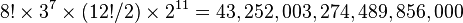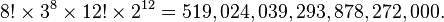## Saturday, September 07, 2013

### erno rubik's magic cube...,

wikipedia | Permutations: The original (3×3×3) Rubik's Cube has eight corners and twelve edges. There are 8! (40,320) ways to arrange the corner cubes. Seven can be oriented independently, and the orientation of the eighth depends on the preceding seven, giving 37 (2,187) possibilities. There are 12!/2 (239,500,800) ways to arrange the edges, since an even permutation of the corners implies an even permutation of the edges as well. (When arrangements of centres are also permitted, as described below, the rule is that the combined arrangement of corners, edges, and centres must be an even permutation.) Eleven edges can be flipped independently, with the flip of the twelfth depending on the preceding ones, giving 211 (2,048) possibilities.${8! \times 3^7 \times (12!/2) \times 2^{11}} = 43,252,003,274,489,856,000$
which is approximately 43 quintillion.
The puzzle is often advertised as having only "billions" of positions, as the larger numbers are unfamiliar to many. To put this into perspective, if one had as many standard sized Rubik's Cubes as there are permutations, one could cover the Earth's surface 275 times.
The preceding figure is limited to permutations that can be reached solely by turning the sides of the cube. If one considers permutations reached through disassembly of the cube, the number becomes twelve times as large:${8! \times 3^8 \times 12! \times 2^{12}} = 519,024,039,293,878,272,000.$
which is approximately 519 quintillion possible arrangements of the pieces that make up the Cube, but only one in twelve of these are actually solvable. This is because there is no sequence of moves that will swap a single pair of pieces or rotate a single corner or edge cube. Thus there are twelve possible sets of reachable configurations, sometimes called "universes" or "orbits", into which the Cube can be placed by dismantling and reassembling it.

Centre faces: The original Rubik's Cube had no orientation markings on the centre faces (although some carried the words "Rubik's Cube" on the centre square of the white face), and therefore solving it does not require any attention to orienting those faces correctly. However, with marker pens, one could, for example, mark the central squares of an unscrambled Cube with four coloured marks on each edge, each corresponding to the colour of the adjacent face; a cube marked in this way is referred to as a "supercube". Some Cubes have also been produced commercially with markings on all of the squares, such as the Lo Shu magic square or playing card suits. Thus one can nominally solve a Cube yet have the markings on the centres rotated; it then becomes an additional test to solve the centres as well.
Marking the Rubik's Cube's centres increases its difficulty because this expands the set of distinguishable possible configurations. There are 46/2 (2,048) ways to orient the centres, since an even permutation of the corners implies an even number of quarter turns of centres as well. In particular, when the Cube is unscrambled apart from the orientations of the central squares, there will always be an even number of centre squares requiring a quarter turn. Thus orientations of centres increases the total number of possible Cube permutations from 43,252,003,274,489,856,000 (4.3×1019) to 88,580,102,706,155,225,088,000 (8.9×1022).
When turning a cube over is considered to be a change in permutation then we must also count arrangements of the centre faces. Nominally there are 6! ways to arrange the six centre faces of the cube, but only 24 of these are achievable without disassembly of the cube. When the orientations of centres are also counted, as above, this increases the total number of possible Cube permutations from 88,580,102,706,155,225,088,000 (8.9×1022) to 2,125,922,464,947,725,402,112,000 (2.1×1024).

Algorithms: In Rubik's cubers' parlance, a memorised sequence of moves that has a desired effect on the cube, is called an algorithm. This terminology is derived from the mathematical use of algorithm, meaning a list of well-defined instructions for performing a task from a given initial state, through well-defined successive states, to a desired end-state. Each method of solving the Rubik's Cube employs its own set of algorithms, together with descriptions of what effect the algorithm has, and when it can be used to bring the cube closer to being solved.
Many algorithms are designed to transform only a small part of the cube without interfering with other parts that have already been solved, so that they can be applied repeatedly to different parts of the cube until the whole is solved. For example, there are well-known algorithms for cycling three corners without changing the rest of the puzzle, or flipping the orientation of a pair of edges while leaving the others intact.
Some algorithms do have a certain desired effect on the cube (for example, swapping two corners) but may also have the side-effect of changing other parts of the cube (such as permuting some edges). Such algorithms are often simpler than the ones without side-effects, and are employed early on in the solution when most of the puzzle has not yet been solved and the side-effects are not important. Most are long and difficult to memorize. Towards the end of the solution, the more specific (and usually more complicated) algorithms are used instead.

Relevance and application of mathematical group theory: Rubik's Cube lends itself to the application of mathematical group theory, which has been helpful for deducing certain algorithms - in particular, those which have a commutator structure, namely XYX−1Y−1 (where X and Y are specific moves or move-sequences and X−1 and Y−1 are their respective inverses), or a conjugate structure, namely XYX−1, often referred to by speedcubers colloquially as a "setup move". In addition, the fact that there are well-defined subgroups within the Rubik's Cube group, enables the puzzle to be learned and mastered by moving up through various self-contained "levels of Difficulty". For example, one such "level" could involve solving cubes which have been scrambled using only 180-degree turns. These subgroups are the principle underlying the computer cubing methods Thistlethwaite and Kociemba, which solve the cube by further reducing it to another subgroup.